GFG App
Open AppBrowser
Continue

A binary number system is a system of numbers that has a base of 2 and uses only two digits, “0 and 1”. It is one of the four types of number systems and is most commonly employed by computer languages like Java and C++. “Bi” in the word “binary” stands for “two.” Some examples of binary numbers are (11)2, (1110)2, (10101)2, and so on. Here, 2 is the radix of a binary number, and each digit is called a bit. A binary number can be converted into other three number systems, i.e., a decimal number system, octal number system, and hexadecimal number system. In this article, we discuss the arithmetic operations on binary numbers and the conversion formulae to convert binary numbers into other three number systems.

## Binary Formula

To understand the binary number system, we must first comprehend its operation. Binary numbers are popularly used in electronic circuits where logic gates are implemented and also by microcontrollers for logical computing. A binary number uses only two digits, ” 0 and 1,” where every digit is known as a bit. The input given to the computer is converted into binary language first with the help of an assigned ASCII code. Furthermore, the information is converted from binary language to the user language and is presented as the output.

## Arithmetic operation on Binary numbers

The addition of binary numbers is a little different from the normal addition but is quite simple. While performing the addition of two binary numbers, we must add them digit by digit. The addition of two binary numbers is also a binary number. Have a look at the table given below to understand the addition of binary numbers.

### Binary Subtraction

While performing the subtraction of two binary numbers, we must subtract them digit by digit. Here, there is no carry as in the addition of binary numbers, but a borrow is needed when we subtract a higher digit from a smaller digit. Have a look at the table given below to understand the subtraction of binary numbers.

### Binary Multiplication

Binary multiplication is similar to the multiplication of normal numbers. Have a look at the table given below to understand the multiplication of binary numbers.

## Binary to Decimal Formula

A decimal number is a most commonly used number in our everyday lives. The decimal number has a base of 10, and 0 to 9 digits are used to represent it. Some examples of decimal numbers are (14)10, (245)10, (1456)10, etc. Now, to convert a binary number “an-1….a3a2a1a0“, multiply every digit of the given binary number with the powers of 2.

If the binary number with n-digits is B = an-1….a3a2a1a0,

Now the decimal number is D = (an-1 × 2n-1) +…+(a3 × 23) + (a2 × 22) + (a1 × 21) + (a0 × 20).

Example: Convert the binary number (1101011)2 to decimal.

Solution:

The given binary number is (1101011)2.

(11001)2 = (1 × 26) + (1 × 25) + (0 × 24) + (1 × 23) + (0 × 22) + (1 × 21) + (1 × 20)

= 64 + 32 + 0 + 8 + 0 + 2 + 1 = 107

Therefore, the binary number (1101011)2 is equal to the decimal number (107)10.

## Decimal to Binary Formula

A decimal number can be converted into a binary number by dividing the given number by 2 continuously till we get the quotient as 1 and write the numbers from downwards to upwards.

Example: Convert the decimal number (39)10 into binary.

Solution:

To convert the (39)10 into a binary number, we have to divide 39 continuously by 2 until we obtain the quotient of 1.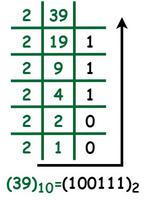Thus, (39)10 in the binary number system is (100111)2.

## Binary to Octal Formula

An octal number is a system of numbers that has a base of 8 and uses digits from 0 to 7. The octal number system was initially used as a computer programming language at an early age. In the octal number system, after 7, the numbers start from 10 to 17, then 20 to 27, and so on. They do not contain digits such as 8 and 9. We have two types of methods to convert a binary number into an octal number, i.e., by converting binary to octal directly, and the other one is converting binary to decimal, and then converting the obtained decimal into octal.

For example, convert (10110110)2 to octal.

Solution:

Method 1:

Step 1: Starting at the right end, divide the given binary number into a pair of three digits.

10-110-110

Step 2: We can notice that the first group doesn’t have three digits. So add zeros on the left. Now, substitute the value of the octal number into it.

010-110-110

(010)2 = (2)8

(110)2 = (6)8

(110)2 = (6)8

Step 3: Now, combine all digits.

010-110-110 = 2-6-6 = 266

Therefore, the binary number (10110110)2 in the octal system is 266.

Method 2:

It is a long process as we have to perform two conversions, i.e., from binary to decimal and again from decimal to octal.

Step 1: Converting the binary number (10110110)2 to decimal

(1 × 27) + (0 × 26) + (1 × 25) + (1 × 24) + (0 × 23) + (1 × 22) + (1 × 21) + (0 × 20)

= 128 + 0 + 32 + 16 + 0 + 4 + 2 + 0 = (182)10

Step 2: Now, divide the obtained decimal number 8.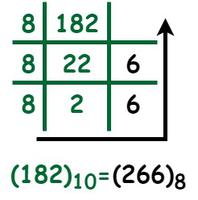Therefore, the binary number (10110110)2 in the octal system is 266.

A hexadecimal number system is a system of numbers that has a base of 16 and uses numbers from 0 to 7 and alphabets from A to F, where the alphabets from A to F represent the numbers 10 to 15 of the decimal number system. Some examples of the hexadecimal number system are AF16, 7BF, B2A1, etc. We have two types of methods to convert a binary number into a hexadecimal number, i.e., by converting binary to hexadecimal directly, and the other one is converting binary to decimal, and then converting the obtained decimal into hexadecimal.

For example, convert (1101010)2 to hexadecimal.

Solution:

Method 1:

Step 1: Starting at the right end, divide the given binary number into a pair of four digits.

1101010 ⇒ 110-1010

Step 2: We can notice that the first group doesn’t have four digits. So add zeros on the left. Now, substitute the value of the hexadecimal number into it.

110-1010 ⇒ 0110-1010

(0110)2 = (6)16

(1010)2 = (A)16

Step 3: Now, combine all digits.

0110-1010 = 6 – A = (6A)16

Thus, the binary number (1101010)2 in the hexadecimal system is 6A.

Method 2:

It is a long process as we have to perform two conversions, i.e., from binary to decimal and again from decimal to hexadecimal.

Step 1: Converting the binary number (1101010)2  to decimal

(1 × 26) + (1 × 25) + (0 × 24) + (1 × 23) + (0 × 22) + (1 × 21) + (0 × 20)

= 64 + 32 + 0 + 8 + 0 + 2 + 0 = (106)10

Step 2:  Now, divide the obtained decimal number by 16.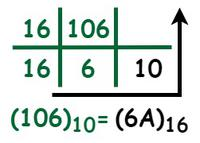We know that in the hexadecimal system 10 is equal to A. Thus, the hexadecimal number is 6A.

Therefore, the binary number (1101010)2 in the hexadecimal system is 6A.

## Solved Examples on Binary Formula

Example 1: Convert the decimal number (73)10 into binary.

Solution:

To convert the (73)10 into a binary number, we have to divide it continuously by 2 until we obtain the quotient of 1.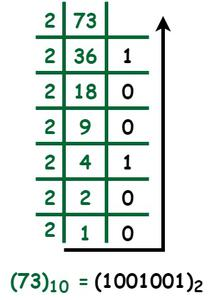Thus, (73)10 in the binary number system is (1001001)2.

Example 2: Convert the binary number (110010101)2 to octal.

Solution:

To convert the binary number into octal, first, we have to divide the given binary number into a pair of three digits, starting from the right end. Now, substitute the value of the octal number into it.

110010101 ⇒ 110 – 010 – 101

6 – 2 – 5 = 625

Therefore, the binary number (110010101)2 in the octal system is 625.

Example 3: Convert the binary number (10110110)2 to hexadecimal.

Solution:

To convert the binary number into hexadecimal, we first have to divide the given binary number into a pair of four digits, starting from the right end. Now, substitute the value of the hexadecimal number into it.

10110110 ⇒ 1011 – 0110

(1011)2 = (B)16

(0110)2 = (6)16

So, (10110110)2 = (B6)16

Therefore, the binary number (10110110)2 in the hexadecimal system is B6.

Example 4: Add (1011010)2 and (1101011)2.

Solution: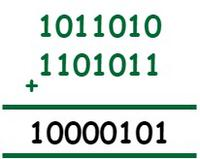Hence, (1011010)2 + (1011010)2 =  (1000101)2.

Example 5: Multiply (110)2 and (101)2.

Solution: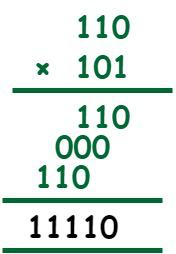Thus, (110)2 × (101)2 = (11110)2.

## FAQs on Binary Formula

Question 1: Define a binary number system.

A binary number system is a system of numbers that has a base of 2 and uses only two digits, “0 and 1”. It is one of the four types of number systems and is most commonly employed by computer languages like Java and C++. “Bi” in the word “binary” stands for “two.” Some examples of binary numbers are (11)2, (1110)2, (10101)2, and so on.

Question 2: What is a formula to convert a binary number into a decimal number?

To convert a binary number “an-1….a3a2a1a0“, multiply every digit of the given binary number with the powers of 2.

If the binary number with n-digits is B = an-1….a3a2a1a0,

Now the decimal number is D = (an-1 × 2n-1) +…+(a3 × 23) + (a2 × 22) + (a1 × 21) + (a0 × 20).

Question 3: What is the formula to convert a binary number into a hexadecimal number?

We have two types of methods to convert a binary number into a hexadecimal number, i.e., by converting binary to hexadecimal directly, and the other one is converting binary to decimal, and then converting the obtained decimal into hexadecimal. To convert the binary number into hexadecimal, we first have to divide the given binary number into a pair of four digits, starting from the right end. Now, substitute the value of the hexadecimal number into it.

Question 4:What is the formula to convert a binary number into an octal number?